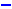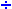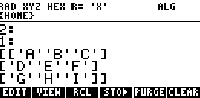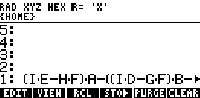Matrices

 Matrices Keyboard access (HP49) Keyboard access (HP48) [MTH] [MATR] Keyboard access (HP49)[Matrices]

Matrices are represented in HP48 and HP49 with brackets [ ],
and as lines x colums.
Inside [ ] we always have a line of the matrix.

Example:
 3 x 1 matrix (3 lines x 1 column ) [    ] 1 x 3 matrix (1 line x 3 columns ) [ [1 2 3 ] ] 4 x 3 matrix (4 lines x 3 column ) [ [1 1 1 ] [2 2 2 ] [3 3 3 ] [4 4 4 ] ] 2 x 3 matrix (2 line x 3 columns ) [ [ 1 2 3 ] [ 4 5 6 ] ]

To find the size of a matrix put it on the stack,
type SIZE and press [ENTER].

Example:
[[ 1 2 3]
[2 2 2 ]]
SIZE

Result: size {2 3}
A matrix with 2 lines and 3 columns.

Operation With Matrices

 1)    + Sums Matrices You can sum only matrices with the same size. If you try sum matrices with different size you will have an invalid dimension error. Example: Put matrices in the levels 1 and 2 and press + [ [2 3] [5 1] ] [ [4 6] [3 2] ] + Result: [ [ 6 9 ]   [ 8 3 ] ] For matrices you can get the same result if you use ADD isntead of + The same commands are also used with lists, however with lists the operator + works different of the operator ADD. 2)Subtracts matrices You can only subtracts matrices with the same size. If sizes are different it will returns invalid dimension error Example: Subtract a matrix in level 1 from a matrix in level 2 [ [2 3] [5 1] ] [ [4 6] [3 2] ]Result: [ [-2 -3] [ 2 -1] ] 3)    x Multiplies Matrices You can Multiply matrices with compatible size. An invalid dimension error will be displayed at status area if the size is not compatible. We know from Math that a matrix A {lines-a columns-a} just can be multiplyed by a matrix B {lines-b columns-b} if the numbers of columns of A is equal the numbers of lines of B, i.e. columns-a = lines-b . The new matrix will have size: {lines-a columns-b} Example: [[1 2 3][4 5 6]]           size {2 3}    ( 2 lines x 3 columns matrix ) [[1 4][2 5][3 6]]           size {3 2}    ( 3 lines x 2 columns matrix ) x Result: [ [14 32]    [32 77] ] size {2 2} 4)Divide matrices You can only divide a square matrix by other of the same size. To divide square matrices put both square matrices on the stack and pressExample: Divede the matrices: [[1 2 ][4 5 ]] size {2 2} [[1 4][2 5]] size {2 2} In HP calculator: [ [1 2 ] [4 5 ] ] [ [1 4] [2 5] ]Result: [[ 3.66666... 3.333333...] [ -.66666... -.333333...]] It is the same that [ [1 2 ][4 5 ] ] [ [1 4][2 5] ] [ 1/X ] [SWAP] x 5) x K Multiplies a Matrix by a constant Put the matrix in level 2 and the constant in level 1 and press x. Example: [[1 2 ][4 5 ]] 5 x Result: [ [5 10]  [20 25] ] The same is also valid forExample: [ [1 2 ] [4 5 ] ] 5Result: [ [.2 .4]  [.8 1] ] 6) 1 / X Inver Matrices To invert a matrix it must 1- be a square matrix. 2- have determinant different of zero. To invert the matrix put it on the stack an press [1/X] Example: [ [5 2 ] [4 8] ] [1/X] Result: [ [.25 -.0625]   [-.125 .15625] ] 7) DET Calculates the determinant of a matrix You just can calculate determinant of a square matrices. To calculate the determinant put the matrix on the stack, type DET and press [ENTER]. Example: [ [4 5 6][9 6 3][-5 8 7] ] DET [ENTER] Result: 294 8) TRN Transpose a Matrix TRN change lines for colums, i.e. transpose a matrix. Example: [ [1 2 3 ] [4 5 6] ] TRN Result: [ [1 4 ]   [2 5]   [3 6] ] 9) RANM Generates a Random matrix Example: {2 3} RANM Result: [ [-1 0 7]  [2 -9 -4] ] Note: the random matrix generated can be, of course, numericaly different of the one in this example. 10) IDN Creates an Identity matrix Example: 3 IDN Result: [ [1 0 0]  [0 1 0]  [0 0 1] ] 11) TRACE Trace calculate the sum of the main diagonal of a square matrix. Example: [ [4 8 9]  [3 6 7]  [2 3 5] ] TRACE Result:15 12) Power Power of a matrix You can calculate the result of the matrix A at the power n using [ENTER] and x Example: On the stack type, [[4 8 9]  [3 6 7]  [2 3 5]] Pressing [ENTER] three times you will get: [ [4 8 9]   [3 6 7]   [2 3 5] ] [ [4 8 9]   [3 6 7]   [2 3 5] ] [ [4 8 9]   [3 6 7]   [2 3 5] ] Press now two times x Result: [ [827 1517 1956]  [627 1150 1483]  [383 702 906] ] That is the matrix at the 3rd power.
Symbolic Matrices in HP49
HP49 has a new feature for matrices.
It is able to solve symbolic matrices.

Example:

Calculate the determinant of a symbolic matrix,

 Set operating mode to RPN 1) Press [MODE] and [+/-] (the W key) 2) Type the symbolic matrix3) Press [ENTER] to put it in the stack 4) Type DET    and press [ENTER] other time.5) It will show a string like ((I.E-H.F).A - (I.D - G.F).B.... in the stack and it is the result itself. Press [ VIEW ] for a best view.Luck sometimes visits a fool, but it never sits down with him. -German Proverb